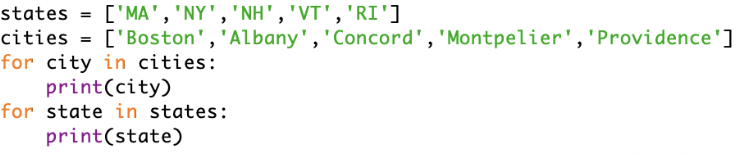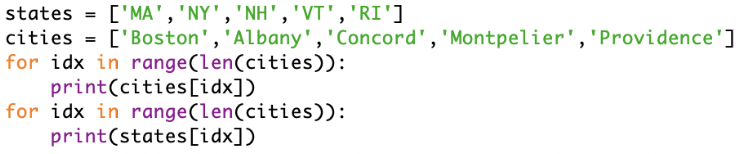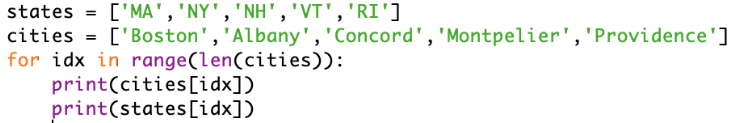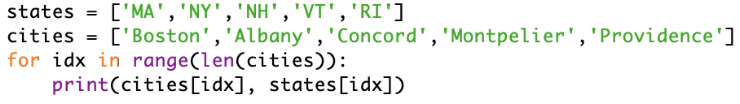Loops checkup #2
This is not a graded quiz: it's for you and me to see which aspects of loops we need to practice more.
What will this code print (assume / means new line in the answers): *
1 pointWhat will this code print (assume / means new line in the answers): *
1 pointWhat will this code print (assume / means new line in the answers): *
1 pointWhat will this code print (assume / means new line in the answers): *
1 pointThis content is neither created nor endorsed by Google. Report Abuse - Terms of Service